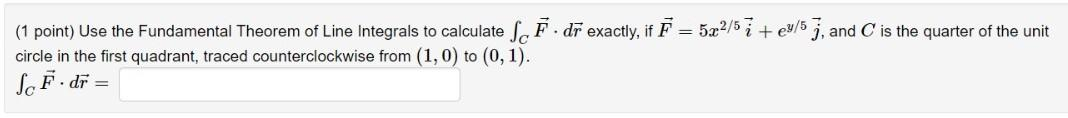### Create an Account

Already have account?

### Forgot Your Password ?

Home / Questions / (1 point) Use the Fundamental Theorem of Line Integrals to calculate So F. dr exactly, if ...

# (1 point) Use the Fundamental Theorem of Line Integrals to calculate So F. dr exactly, if F = 522/5 7 +e3/5 7, and is the quarter of the unit circle in the first quadrant, traced counterclockwise from

(1 point) Use the Fundamental Theorem of Line Integrals to calculate So F. dr exactly, if F = 522/5 7 +e3/5 7, and is the quarter of the unit circle in the first quadrant, traced counterclockwise from (1,0) to (0,1). SF. dr =Apr 15 2021 View more View Less

#### Answer (Solved)Subscribe To Get Solution